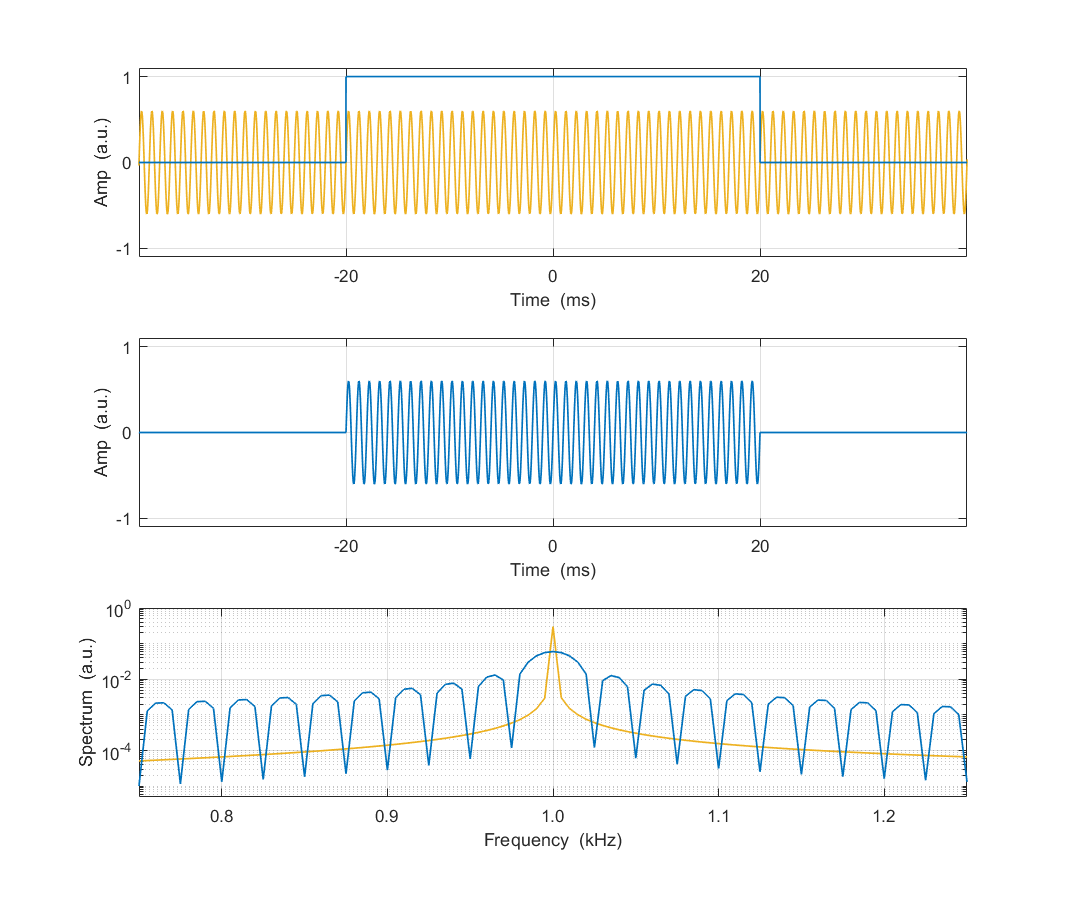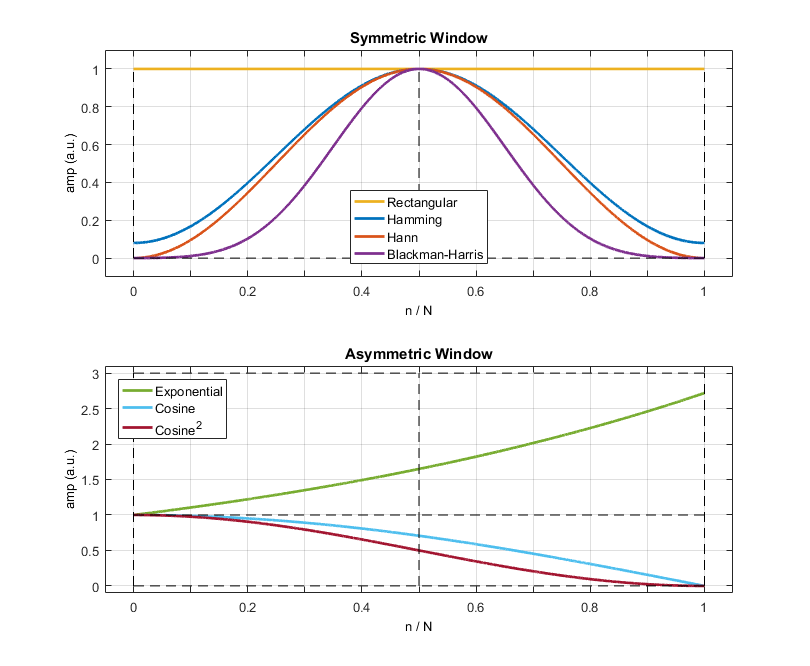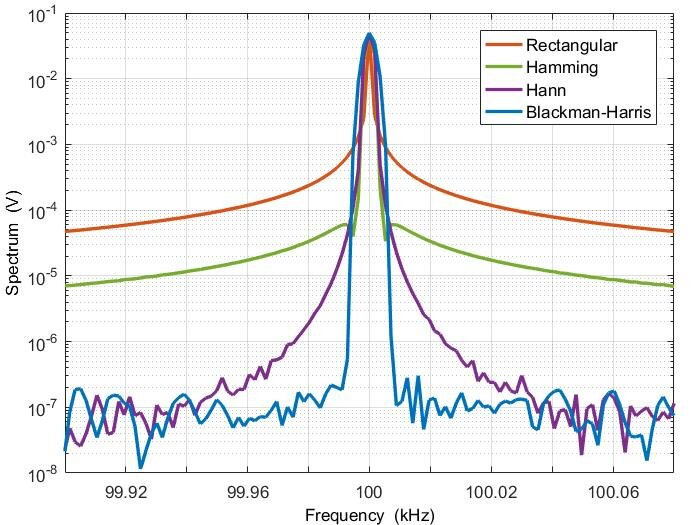# How to Control Spectral Leakage with Window Functions in LabOne

November 25, 2021 by Mehdi Alem

## Introduction

Spectral characterization of signals and systems is required in a broad range of applications in physics and engineering. Analyzing a signal in the frequency domain reveals valuable information about the signal behavior which is not necessarily obtainable from its time-domain analysis. Therefore, it is crucial for all test and measurement (T&M) instruments to provide a reliable and efficient toolset for spectral analysis of signals. A ubiquitous tool to convert a measured temporal signal to its frequency-domain representation is the Fourier transform (FT). Its digital version called discrete Fourier transform (DFT) is widely implemented in digital T&M systems using the fast Fourier transform (FFT) algorithm. Due to some limitations, however, spectral leakage appears to be a fundamental problem with this approach. In this post, we investigate spectral leakage when obtaining signal spectrum and how it can be mitigated using window functions. To avoid measurement inaccuracy, we then show how to compensate for the impact of window functions on the spectral density of signals.

## Spectral Leakage

When taking the Fourier transform of signal, one implicitly assumes that the temporal signal is available for the entire time axis and the whole signal contributes to its Fourier transform. However, it is either impossible or computationally expensive to take the entire signal into account. Instead, we only select a portion of the signal for our spectral analysis which is like slicing the signal with a rectangular window as shown in Figure 1.Figure 1: Demonstration of spectral leakage due to windowing. The top plot shows a sine signal of 1 kHz along with a window of length 40 ms. Middle plot displays the signal after applying the window function. The outcome includes 40 cycles of the sine signal. The bottom plot compares the spectrum of actual signal (orange) and windowed signal (blue) clearly displaying the spectral leakage due to the window function.

Suppose we are interested to obtain the spectrum of a sine signal shown in the top plot of Figure 1 to discover its frequency. In practice, we take a slice of the signal which is equivalent to applying a rectangular window to the signal as depicted in the middle plot of Figure 1 and then we calculate the Fourier transform of the sliced signal. The bottom plot of Figure 1 compares the spectrum of an ideal sine signal with that of the windowed signal. We expect a narrow spectral line (ideally an impulse function) for the sine spectrum. However, we obtain a broadened spectrum with multiple lobes around the signal frequency due to the limited duration of the signal induced by the window function. This phenomenon is called spectral leakage because the signal energy from a frequency component has leaked to another frequency component which does not exist in the original signal. Spectral leakage degrades the amplitude accuracy and reduces the frequency resolution of the measurement. One way to avoid spectral leakage is to properly choose the sampling rate and acquisition time based on signal characteristic as demonstrated in this blog post. However, those parameters are not often as flexible as required to avoid spectral leakage. A more prevalent approach to mitigate spectral leakage is to apply tailored window functions to the signal which will be discussed below.

## Window Function

Selecting a segment of a signal to calculate its Fourier transform is equivalent to applying a rectangular window to the signal as shown in Figure 1. Such a window function results in the undesired phenomenon of spectral leakage. Nevertheless, by tapering the shape of the window, it is possible to tailor the spectral leakage and mitigate its consequences when taking the DFT of the signal segment . There are two types of window functions which serve different purposes:

• Symmetric window
• Asymmetric window

Symmetric window functions have a symmetric shape around their mid point, and they are widely used for handling spectral leakage in frequency-domain measurement; while asymmetric windows do not have a symmetry line in the middle, and they are mostly used to mitigate spectral leakage when time-domain measurement techniques such as the ring-down method are employed. Figure 2 depicts the symmetric and asymmetric window functions available in LabOne®, the instrument control and data acquisition software of Zurich Instruments.Figure 2: Symmetric and asymmetric window functions used for spectral and temporal measurements in LabOne, respectively.

Here, we focus on spectral measurements and thus only symmetric window functions are discussed. As we see from the top plot of Figure 2, there are a few window functions available to taper the signal segment: the main question is what the best choice of window function is. Of course, the answer depends on the application requirements, but there are two main criteria and, therefore, two sets of window functions:

• High dynamic range to resolve a weak frequency component from a strong one
• High frequency resolution to resolve two near frequency components with similar strength

There is a trade-off between these two criteria and a window function which is good for one criterion usually lacks the other one. An example of window with a high dynamic range available in LabOne is Blackman Harris, and an extreme case of high frequency resolution is the rectangular window. A balanced trade-off between these two criteria is achieved via Hann and Hamming window functions: that’s why LabOne uses Hann as its default window function for spectrum measurements. Table 1 shows the mathematical definition of window functions used in LabOne for spectrum (symmetric) and ring-down (asymmetric) measurements. Here, $$N$$ determines the window length where $$0\leq n \leq N$$.

Table 1: List of window functions implemented in LabOne.
Window Formula Property
Rectangular (boxcar) $$1$$ Symmetric
Hamming $$0.54 - 0.46\cos(\frac{2\pi n}{N})$$ Symmetric
Hann $$0.5 - 0.5\cos(\frac{2\pi n}{N})$$ Symmetric
Blackman-Harris  $$0.35875 - 0.48829\cos(\frac{2\pi n}{N}) + 0.14128\cos(\frac{4\pi n}{N}) - 0.01168\cos(\frac{6\pi n}{N})$$ Symmetric
Exponential $$\exp(\frac{n}{N})$$ Asymmetric
Cosine $$\cos(\frac{\pi n}{2N})$$ Asymmetric
Cosine squared  $$\cos^2(\frac{\pi n}{2N})$$ Asymmetric

These window functions are available in the settings of the Scope, Data Acquisition (DAQ) and Spectrum tools of LabOne. To demonstrate the impact of window functions on a real signal, we measure the spectrum of a sine signal at 100 kHz using the scope module of LabOne. In addition to the temporal measurement, this module displays the frequency-domain representation of the acquired signal for different window functions. Figure 3 shows the outcome of this measurement for the 4 symmetric window functions offered by LabOne.Figure 3: Spectrum of a 100-kHz signal measured with the MFLI Lock-in Amplifier and acquired by the Scope module of LabOne for 4 window functions: Rectangular, Hamming, Hann, and Blackman-Harris.

The impact of window functions on the signal spectrum is clearly shown in Figure 3, in particular the spectral leakage which changes substantially when changing the type of window functions. In the vicinity of signal frequency, spectral broadening increases when moving from rectangular to Hamming, Hann, and Blackman-Harris windows, respectively. By contrast, when away from the center frequency, leakage increases when moving in the opposite direction from Blackman-Harris to Hann, Hamming and rectangular functions.

## Spectral Density

In many frequency-domain measurement techniques, the goal is to obtain the spectral density of the signals or noise sources involved in the system under test. Whether it is amplitude or power spectral density, one measurement approach is to acquire the time-domain signal, calculate its Fourier transform, and divide it by the frequency resolution of the measurement instrument. Without applying a window function or actually with the rectangular window, the frequency resolution is simply obtained by $$\Delta f = \frac{F_s}{N}$$, where $$F_s$$ is the sampling frequency and $$N$$ is the number of acquired samples. By applying a window, however, the frequency resolution is modified by the equivalent noise bandwidth (ENBW) of the corresponding window function. In other words, the equivalent frequency resolution is given by $$c_w\Delta f$$, where the ENBW correction factor $$c_w$$ is obtained from the following expression:

$c_w = \frac{\int{|w(t)|^2 dt}}{|\int{w(t)dt}|^2}$

Table 2 provides the ENBW correction factor of the 4 symmetric window functions available in LabOne.

Table 2: Equivalent noise bandwidth of symmetric window functions.
Window $$c_w$$
Rectangular $$1.00000$$
Hamming $$1.36283$$
Hann $$1.50000$$
Blackman-Harris $$2.00435$$

Now, we can precisely define the power spectral density (PSD) of the voltage signal $$x$$ in $$\frac{\text{V}^2}{\text{Hz}}$$ as follows.

$S_x = \frac{|\text{FFT}(x)|^2}{c_w\Delta f}$

The amplitude spectral density given in $$\frac{\text{V}}{\sqrt{\text{Hz}}}$$ is simply obtained by taking the square root of PSD. As a result, the amplitude spectral density of the signal $$x$$ acquired with frequency difference $$\Delta f$$ and multiplied by window function $$w$$ before FFT calculation is expressed as

$\sqrt{S_x} = \frac{|\text{FFT}(x)|}{\sqrt{c_w\Delta f}}$

The LabOne graphical user interface takes the ENBW correction coefficient $$c_w$$ into account when displaying the spectral density. However, when recording the signal spectrum from a module history or when using one of LabOne APIs, one can only record the signal spectrum and not its spectral density. To obtain the spectral density of the signal, one should apply the above expression to the recorded signal in post-processing stages. The recorded files include the signal spectrum, frequency resolution, and the correction factor of the selected window function. Using this information one can obtain the spectral density of the signal from the signal spectrum as demonstrated in the following MATLAB® code snippet. Similar codes can be written in other languages such as Python and C.

%%%%%%%%%%%%%%%%%%%%%%%%%%%%%%%%%%%%%%%%%%%%%%%%%%%%%%%%%%%%%%%%%%

% Serial number of instrument
device = 'dev3305';

% Load measured spectrum from file 'spectrum_00000.mat'
data = struct.(device).demods.sample_xiy_fft_abs_avg{1,1};

% Extract frequency information

% Calculate frequency range
freq = freq_delta*(0:double(freq_num)-1) + freq_offset;

% Extract spectrum of signal from loaded data
spectrum = data.value(1,:);

% Extract correction factor of window function

% Calculate spectral density
spectral_density = spectrum / sqrt(freq_delta*factor_nep_window);

%%%%%%%%%%%%%%%%%%%%%%%%%%%%%%%%%%%%%%%%%%%%%%%%%%%%%%%%%%%%%%%%%%

## Conclusions

Window functions are widely used for managing spectral leakage when calculating the Fourier transform of a signal. The LabOne software offers 4 symmetric window functions, namely rectangular, Hamming, Hann, and Blackman-Harris. Depending on the requirements, users can select any of them to handle spectral leakage in their spectrum measurement. Care should be taken when extracting the spectral density of a signal from its Fourier transform, because the window function modifies the equivalent noise bandwidth of the measurement process.

## References

1. Wikipedia: Window function.
2. Mathuranathan Viswanathan, Equivalent noise bandwidth (ENBW) of window functions, GaussianWaves, 2020.